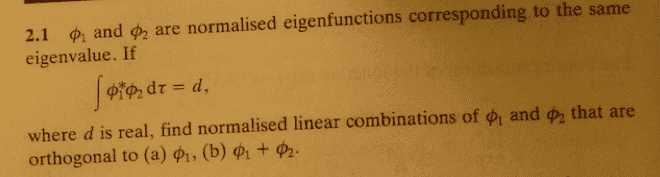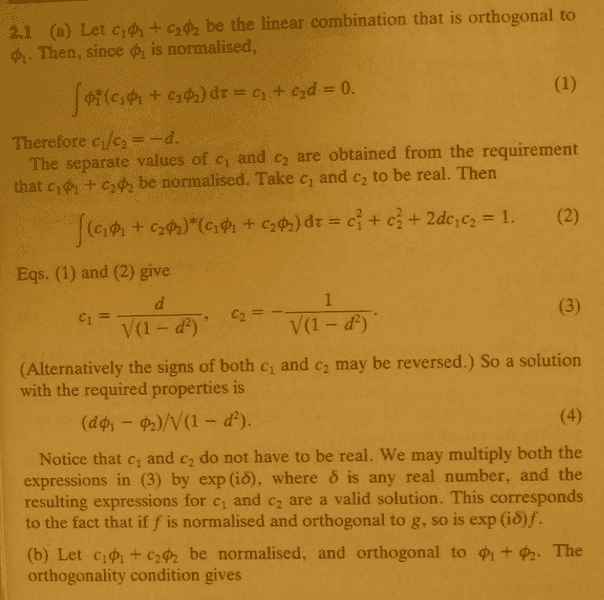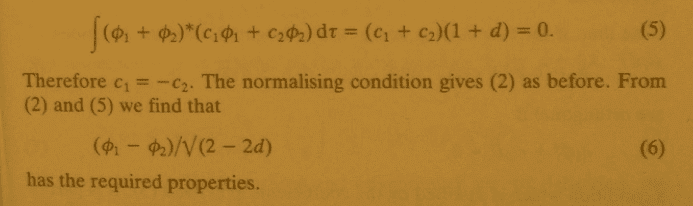# Find normalised linear combinations that are orthogonal

g.lemaitre

## Homework Statement

I'm a little weary of posting this in this forum. If I post it in the math section it will be answered in about 30 min whereas here it might take about 5 hours, but we'll see.## The Attempt at a Solution

Number one, I'm not exactly sure how they get from
$$\int \phi_1^* (c_1\phi_1 + c_2\phi_2)d\tau = c_1 + c_2 d$$

I think it's because

$$\phi^*\phi = 1$$ but I'm not sure.
Number two, I don't understand the following step:
$$\int (c_1\phi_1 + c_2 phi_2)*(c_1 \phi_1 + c_2\phi_2)d\tau = c_1^2 + c_2^2 + 2dc_1c_2$$

why does $\phi$ disappear?
I figure that it must have something to do with the fact that $\phi_1$ is orthogonal which means it = 0
Number three, I can't get step 3. I put the equations as follows:
$$c_1 + c_2d = 0 c_1^2 + c_2^2 + 2 dc_1c_2 = 1 c_1 = -c_2d$$
therefore
$$(-c_2d)^2 + c_2^2 + 2d(-c_2d)c_2 = 1 (-c_2d)^2 + c_2^2 - 2(c_2d)^2 = 1$$
And then I can go no further.
Number four, I don't understand how
$$\int (\phi_1 + \phi_2)*(c_1\phi_1 + c_2\phi_2)d\tau$$

simplifies to
$$(c_1 + c_2)(1+d)$$
Number five, what do they mean by 2 and 5 gives
$$\frac{(\phi_1 - \phi_2)}{\sqrt{2-2d)}}$$
As you can see I'm real clueless with regards to this stuff. I've got a private tutor lined up but I won't be able to meet with him until sometime next week.

Last edited by a moderator:

Aimless
From the first sentence of the problem: "$\phi_1$ and $\phi_2$ are normalized eigenfunctions..."

What is the definition of normalized? Specifically, how does the fact that $\phi_1$ and $\phi_2$ are normalized relate to the integrals $\int \phi_1^*\phi_1 d\tau$ and $\int \phi_2^*\phi_2 d\tau$?

Answer that question and that should tell you everything you need to know to work through the derivation.

g.lemaitre
Normalized means = 1. I already knew that. Still clueless.

Aimless
What, specifically, is equal to 1?

Edit: Hint - Your statement in the original post that $\phi_1^* \phi_1 = 1$ is not quite correct. What is the correct equation?

Last edited:
g.lemaitre
$$\phi_1 \phi_2$$

Aimless
Not true. Nothing in the problem set up indicates $\phi_1 \phi_2 =1$ in general. The correct relation between the two is $\int \phi_1^* \phi_2 d\tau = d$.

So, I think I'm seeing several issues here that are causing you problems. First, you need to be a lot more careful about the difference between $\phi$ and $\phi^*$. The two are not the same, and which terms have the * and which terms don't matters.

Second, you need to go back and review the definition of normalization. I think you have assumed that it means something that it doesn't mean, and that's why you can't evaluate the integrals above.

Third, slow down, take a bit more time, and don't skip any steps when you are working through the problem. Also, when replying on this thread, be as specific as you can; it'll make it easier to diagnose where your misunderstanding is coming from.

To work through each of the integrals in the derivation above, you need to know three different quantities:

$\int \phi_1^* \phi_1 d\tau = ?$
$\int \phi_2^* \phi_2 d\tau = ?$
$\int \phi_1^* \phi_2 d\tau = ?$

Each of the integrals in the derivation can be broken up into pieces containing those three terms.

g.lemaitre
I think I'll just wait until I meet with that private tutor. My problems are just so overwhelming. I think maybe I zipped through the calc, linear algebra and DE prerequisites too quickly. Maybe I'm trying QM without sufficient knowledge.

Aimless
Actually, the math in this particular derivation is quite simple, once you have all of your definitions straight. That's why I was asking you about what normalization meant. I don't think this particular derivation is beyond you, I just think you need to slow down, take your time with it, and review the basics.

As another hint: for a normalized (1d) wave function in the position representation, $\phi(x)$, the probability of finding the particle between the points $x_1$ and $x_2$ is given by $P[x_1<x<x_2]=\int_{x_1}^{x_2} \phi^*(x) \phi(x) dx$.

If I take $x_1$ to $-\infty$ and $x_2$ to $\infty$, what's the probability of finding the particle between $x_1$ and $x_2$? That is,

$P[-\infty<x<\infty]=\int_{-\infty}^{\infty} \phi^*(x) \phi(x) dx = ?$

Don't try to solve the integral, just use common sense. What's the probability of finding the particle if we search the entirety of the real line?

g.lemaitre
as for the odds of finding a particle between negative infinity and plus infinity it has to be 1, nothing can exist beyond the edge of infinity since there is no edge to infinity. I certainly hope the math is not beyond me.

Aimless
Exactly. $P[-\infty<x<\infty]=\int_{-\infty}^{\infty} \phi^*(x) \phi(x) dx = 1$. That is the definition of what it means for a wave function to be normalized.

So, what does that statement imply for the three integrals needed for this problem?

$\int \phi_1^* \phi_1 d\tau = ?$
$\int \phi_2^* \phi_2 d\tau = ?$
$\int \phi_1^* \phi_2 d\tau = ?$

g.lemaitre
I'm guessing they equal 1.

Aimless
Not all of them. :) One of the three is given to you in the problem. The other two equal 1.

g.lemaitre
$\int \phi_1^* \phi_2d\tau = 0$ because it's orthogonal, right?

Aimless
Nope. Actually, that's the point of the problem. $\phi_1$ and $\phi_2$ are not orthogonal, but rather integrate to a constant, $\int \phi_1^* \phi_2 d\tau = d$. The question is, given that the are not orthogonal, how would you go about constructing orthogonal functions from them.

That is, you are trying to find some $\bar{\phi}_2 \equiv c_1 \phi_1 + c_2 \phi_2$ such that $\int \phi_1^* \bar{\phi}_2 d\tau = 0$.

g.lemaitre
I have to leave now and I will return to this problem after about 20 hours have passed. I appreciate you helping me.